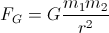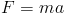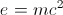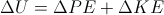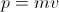# SAT II Physics : Linear Motion Principles

## Example Questions

### Example Question #1 : Linear Motion Principles

A model rocket, launched vertically, travels upwards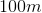and falls to the ground. At what point during flight is the rocket's acceleration greatest?

Just after launch

At the highest point of the flight

Just before landing

The acceleration remains constant throughout the flight

The answer cannot be determined from this information

The acceleration remains constant throughout the flight

Explanation:

Newton's second law tells us that force and acceleration are directly related; if there is an acceleration, then there is also a force. This principle can help conceptualize this question.

While the rocket is in the air, there is only one force acting on it: the force of gravity. We can thus conclude that the acceleration of the rocket is directly related to this force. Since the force on the rocket (the force of gravity) is constant, its acceleration is also constant.

Any object that is in projectile or free-fall motion will experience a constant acceleration due to gravity.

### Example Question #6 : Understanding Newton's Second Law

Which of these is not an example of Newtonian mechanics?